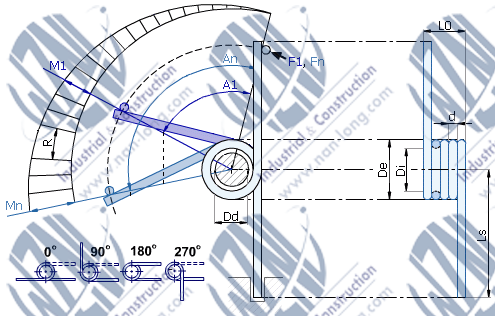# Torsion Spring|ChinaSpring >>

# Torsion Spring#Physical parameters :

• Wire Diameter: This parameter describes the diameter of wire used as material for spring.
• Internal Diameter(Di): Internal diameter of a spring. It can be calculated by subtracting the wire diameter multiplied by 2 from the external diameter of a spring. Internal diameter in torsion springs decreases while the spring is working even up to shaft diameter.
• External Diameter(De): External diameter of a spring. It can be calculated by adding the wire diameter multiplied by 2 to the internal diameter of a spring. External diameter in torsion springs decrease while the spring is working. Tolerance for this parameter is (+-)2% with tolerance (+-) 0.1 mm
• L0 (Free Length): WARNING: Free Length decrease while spring is working. Tolerance for this parameter is (+-)2%(indicative).
• Ls (Leg Length): It is the distance from the center axis of the coil body to the end of the torsion springs' leg. Tolerance for this parameter is (+-)2%(indicative).
• An (Maximum angle): Maximum acceptable rotation angle for the spring in degrees. Tolerance for this parameter is (+-)15 degrees (indicative).
• Fn (Maximum force): Maximum acceptable force that can operate at the end of torsion springs' leg.
• Mn (Maximum torque): Maximum acceptable torque (Newton * mm).
• R (Spring rate): This parameter determines spring's resistance, while it is working. It is measured in Newton * mm / degree.
• A1 & F1 & M1: (angle at torque or force) The following equation calculates the angle as a function of the torque: A1 = M1/R. In order to calculate the torque from the force, we use this equation: M = F*Ls
• Leg position There are 4 types of torsion springs' leg positions: 0, 90 ,180 or 270 degrees (see picture above).
• Helix direction: A right-hand-wound spring loads in a counter-clockwise direction. A left-hand-wound spring loads in a clockwise direction. Both types are available in all sizes.
• Reference Number: All springs are identified by a unique reference: type . (De * 10) . (d * 100) . (N * 100) . For right-hand-wound springs, the type is a letter "D". For left-hand-wound springs, the type is a letter "G". "N" stands for the number of coils. Example: D.028.020.0350 is a right-hand-wound torsion spring with an external diameter of 2.8mm produced from 0.9mm stainless steel wire and having 3.5
• Torsion Spring Pitch: p, the distance between wire centers in adjacent body coils. This dimension is equal to the wire diameter when the body is close wound, where adjacent coils are contacting each other in the free state. However, it is also common to produce torsion springs with pitch in the body to avoid the effects of friction during deflection. In this case it is recommended practice is to specify the number of active coils and a body length rather than pitch.
• Torsion Spring Rate: The change in load per unit of deflection, generally expressed in pounds per degree. Spring rate is determined by the amount of force, in pounds, required to rotate a spring by one degree.